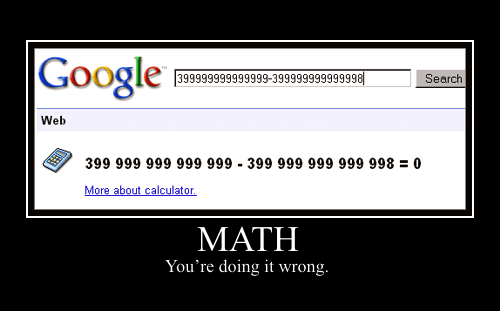# How to Use Google to Solve Simple & Complicated Mathematics

I’m a very lazy students, I love doing things easily without undergoing unnecessary stress. My co-friends called me smart, because I take the easy route most time and come out excellently.Google is my good friend, and the secret of my smartness. For every student to have outstanding results, Google must not be left out since it can direct you to many resources that will help you succeed. Apart from the fact that Google helps to garner the best resources for success, it also possess an inbuilt capabilities that can help with simple and complicated mathematical problems without much ado. It remembered some years back when I was still in high school, my teacher asked us to solve for 50!, I immediately typed it on Google and it brought out the result instantly without using calculator. Click here to verify.

Today, I want to explain how you can easily use your browser and Google to solve for simple and complicated mathematical problems without much ado using Google’s built-in calculator function.

#### 1. Basic Arithmetic

To easily compute mathematical expressions that contains symbols, the following table lists operators that come between the two numbers on which they operate, e.g., to multiply 5 times 6, use 5 * 6.

Operator Function Example
+ Addition 20.99 + 50.50 + 17.25 ]
Subtraction 99 – 28 – 39 ]
* Multiplication 2 * 3 * 4 ]
/ Division 378 / 9 ]
`^` or `**` Exponentiation (raise to a power of) 4^10 ]
or [ 4**10 ]
% of Percent 15% of 93.45 ]
mod or % modulo (the remainder after
division)
15 mod 9 ]
or [ 15 % 9 ]
the `n`th
root of
calculates the `n`th
root
4th root of 16 ];
cube root of 109 ];
square root of 42 ] or
sqrt(42) ]

To easily compute mathematical problems involving constants such as: e, pi, i (the square root of –1), and mathematical functions. The following table lists some of the functions built into Google’s calculator.

Operator Function Example
sin, cos, tan, sec, csc, cot, etc. Trigonometric functions (arguments
are assumed to be in radians)
cos(pi/6) ]; [ cosine(pi/6) ]
arcsin, arccos, arctan, arccsc,
etc.
Inverse trigonometric functions arccos(.5) ]
sinh, cosh, tanh, csch, arsinh,
arccsch, etc.
Hyperbolic functions cosh(6) ]
ln Logarithm base e ln(16) ]
log Logarithm base 10 log(16) ]
lg Logarithm base 2 lg(16) ]
exp Exponential function exp(16) ]
! Factorial 5! ]
choose x choose y calculates the number of
ways of choosing a set of y elements from a set of x distinct elements
5 choose 3 ]

The following table lists just a few of the commonly used mathematical constants known to the calculator function.

Name and description Example
base of the natural system of
logarithms
e ]
pi, the ratio of the circumference
to the diameter of a circle
pi/6 ]
imaginary number, i, which
represents one of the two square roots of -1
i^2 ]
Euler’s constant, gamma e^gamma ]

Hope you enjoyed it? You can also share the ones you know via the comment box below.

1.edoma patrick moses
2.prettypresh
3.Ugare jr.
4.Nkemakonam Ezenwa
5.Kemidean
6.Rohan Mod